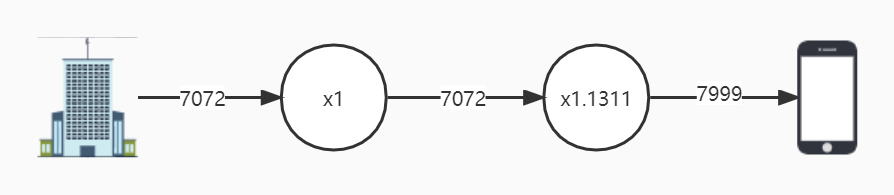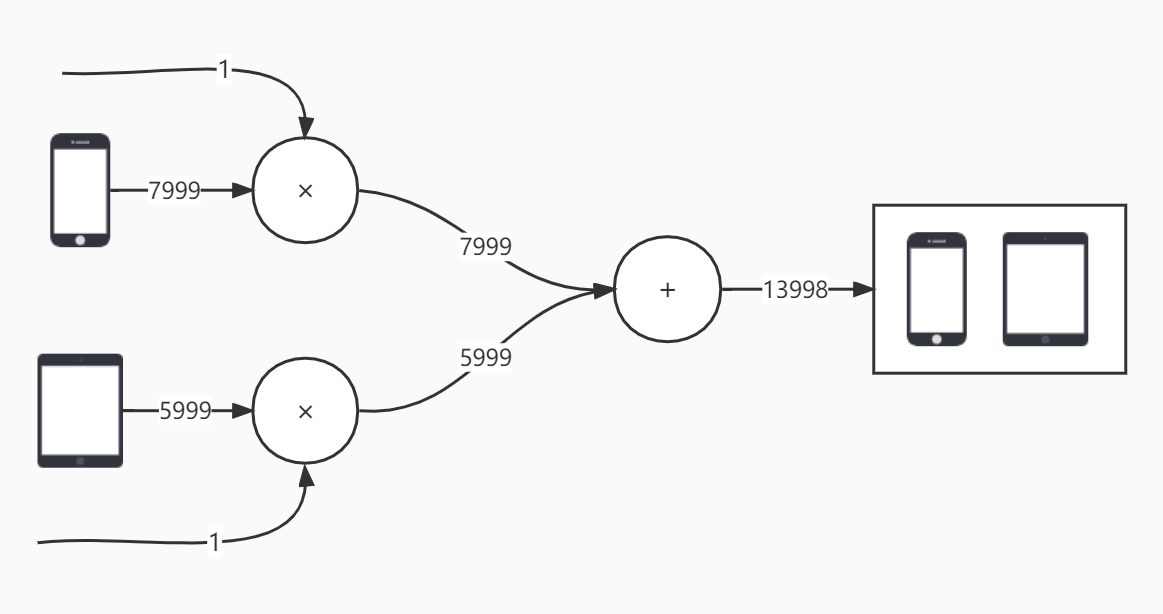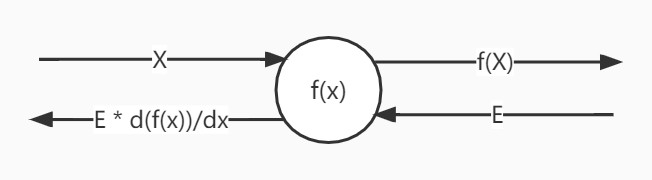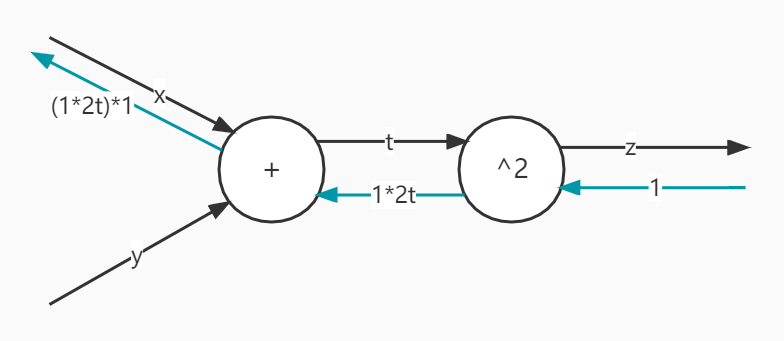# 【深度学习笔记】误差反向传播（1）

【深度学习笔记】神经网络的学习（3）中，我们使用数值微分的方法去训练神经网络，速度特别慢，这篇文章介绍一下误差反向传播，不再使用数值微分的方法，可以快速计算剃度进行参数的更新。

## 前置知识

### 计算图## 误差反向传播

### 反向传播$$z=(x+y)^2$$

$$\frac{\partial z}{\partial t}=\frac{\partial t^2}{\partial t}=2t$$
$$\frac{\partial t}{\partial x}=\frac{\partial (x+y)}{\partial y}=1$$
$$\frac{\partial t}{\partial y}=\frac{\partial (x+y)}{\partial y}=1$$

$$\frac{\partial z}{\partial x}=\frac{\partial z}{\partial t} \frac{\partial t}{\partial x}=2t=2(x+y)$$